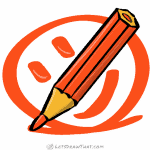# How to Draw a Hexagon: Using Compass and Hand-drawn

Learn how to draw a hexagon in two ways. Construct a precise hexagon using a compass or learn how to draw hexagon from six triangles by hand.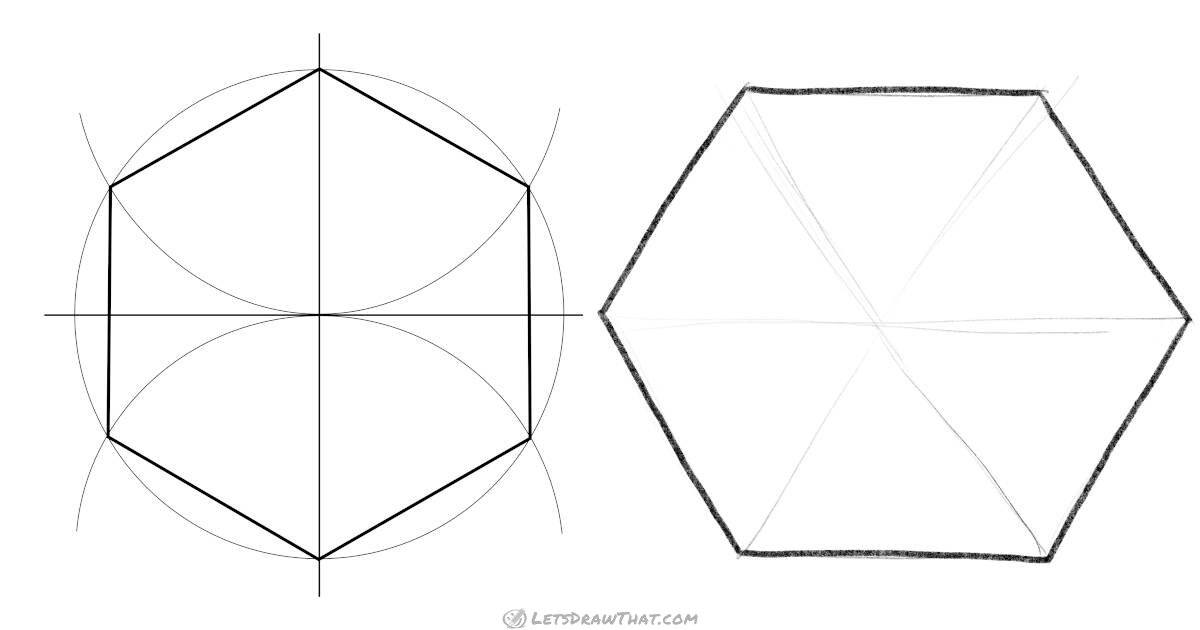The first way how to draw a hexagon is to construct it precisely using a compass and ruler. That way we get a geometrically perfect hexagon.

Our second hexagon is drawn by hand and built up from six triangles of the exact same shape. This is an easy technique how to draw a hexagon with a reasonably regular shape by hand.

Drawing time:
You will need:
pencil
eraser
colour pencils
drawing paper
or
sketch book

## How to draw a hexagon with a compassOur first method of how to draw a hexagon uses a compass and a ruler for precise construction - we get a geometrically perfect shape. All sides of the hexagon are the same length – exactly half of the diameter of the circle it is inscribed in. That makes it easy to split the circle regularly and mark the required points on the circle with a compass. Let’s get into it.

### Hexagon drawing with a compass

#### Step 1: Draw a circle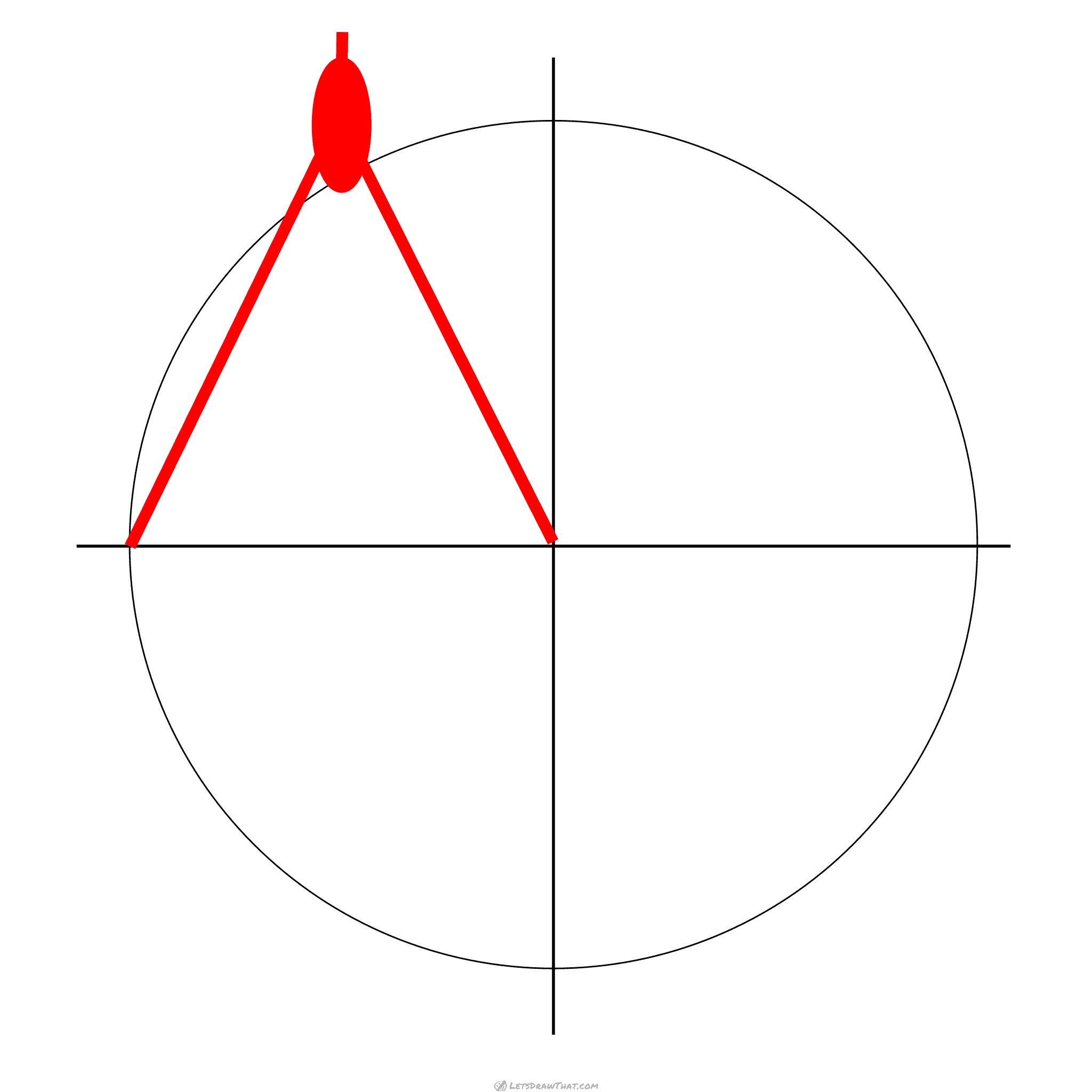First draw a cross – two guidelines of the same length, crossing at a right angle (90 degrees). These will form the base of our construction.

Then take your compass and draw a circle from the centre where the two lines cross.

If you are aiming to construct a hexagon with a given side length, the radius of the circle, i.e. the opening of your compass, is the given length of the side of a completed hexagon.

#### Step 2: Mark the top two corners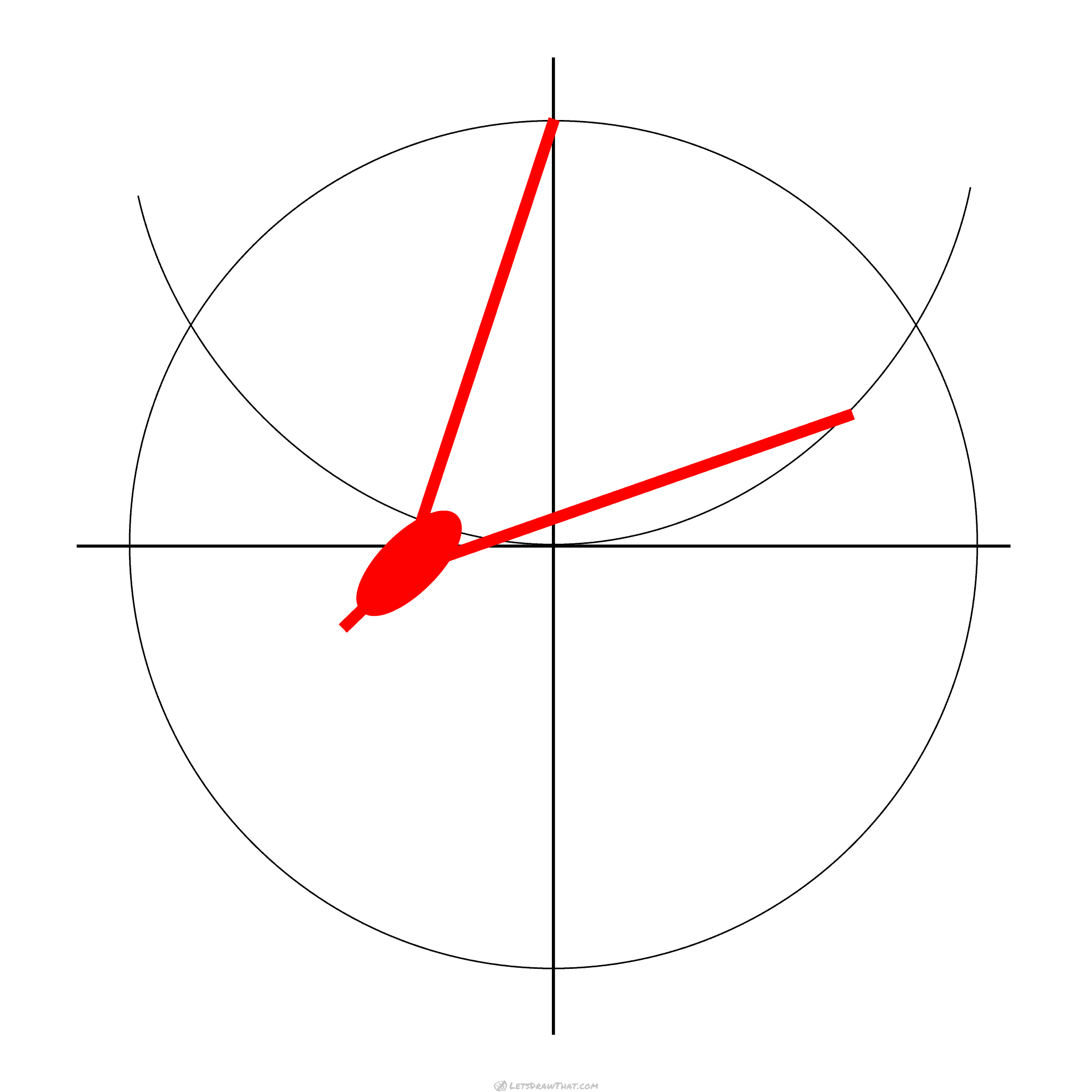Next, we will mark the top two corners. Leave your compass set to the same radius, and draw a semicircle from the top intersection of our circle and the vertical cross line.

If you are aiming to erase all these construction lines later, just mark the spots where the semicircle intersects with the circle.

We have drawn the full half-circle here just to show the fact that we still draw with the same radius, and to make the explanation a bit more obvious.

#### Step 3: Repeat and mark the bottom two corners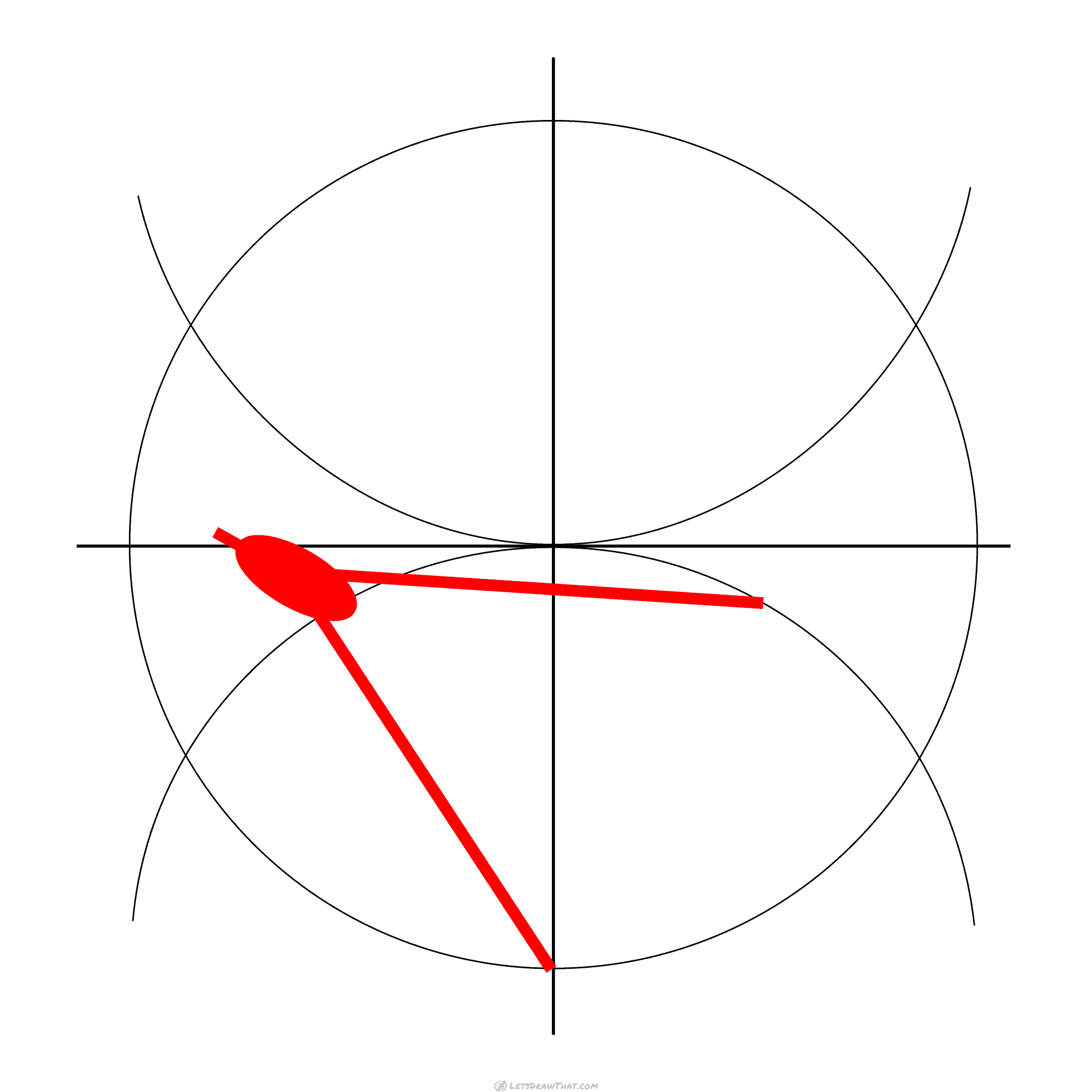We repeat and draw the half-circle with exactly the same radius from the bottom intersection of our circle and the vertical cross line. That marks the remaining two points of our hexagon at the bottom half of the circle.

Again, feel free to just mark the spots, rather than drawing the full semicircle.

#### Step 4: Connect the marked points to draw a hexagon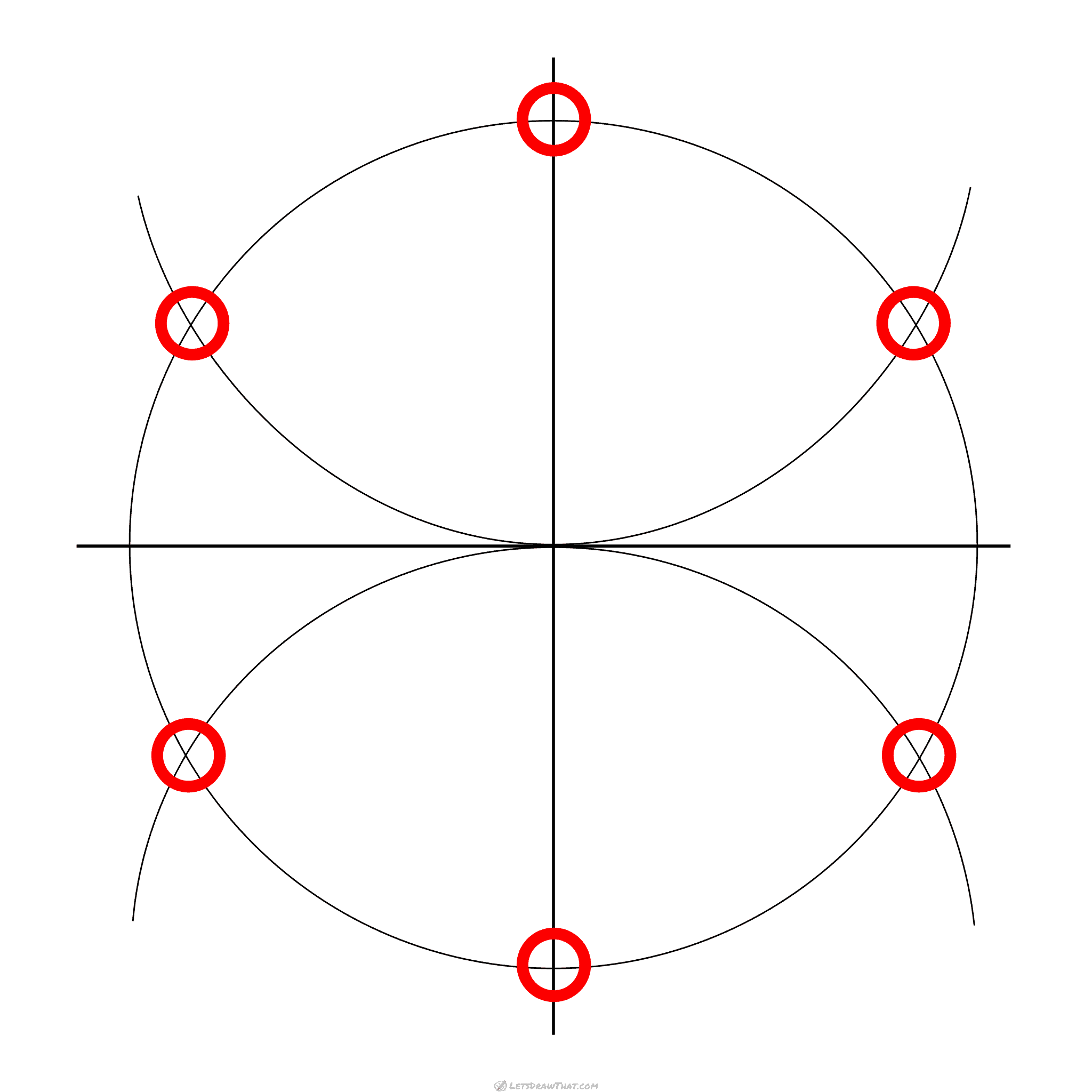On this picture we have highlighted the six intersecting points that will make the corners of our hexagon.

All that is left is to connect them all with straight lines and we have constructed a perfect regular hexagon.

### How to draw a hexagon with a compass: finished drawing

#### How to draw a hexagon with a compass: completed perfect hexagonHere is the complete geometrically perfect hexagon.

If you would want the hexagon to sit on the flat side, rather than stand on its corner, you can use the same technique. Just draw the half circles from the left and right, rather than the top and bottom intersection of the cross and circle.

And finally, if you do not have a compass on hand: You can get pretty close using anything round, such as the top of a glass, to draw your circles. Just try to make the top and bottom semicircle meet as closely as possible to the centre of the full circle.

Now you have learned an easy method of how to draw a hexagon using the compass to construct a perfectly regular hexagon.

Like this tutorial? Share it with others:
Enjoying the geometry? Here is another technical drawing for you: How to draw a cube: 3 different ways and perspectives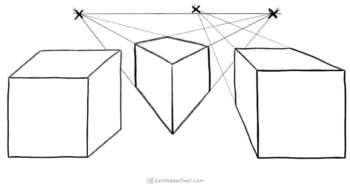#### How to draw a cube: 3 different ways and perspectives

How to draw a cube: learn 3 common ways to show three dimensional cube on a flat paper - simple 3D view and 1 point and 2 point perspective.

## How to draw a hexagon by hand (without a compass)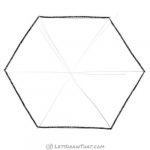In a regular hexagon, a length from the centre to any corner of the hexagon is the same length as any side. Because of this, a regular hexagon can be thought of as being made of six equilateral triangles – triangles with all three sides of the same length. Let’s use that knowledge to learn how to draw a hexagon by hand.

### Drawing hexagon by hand

#### Step 1: Draw a triangle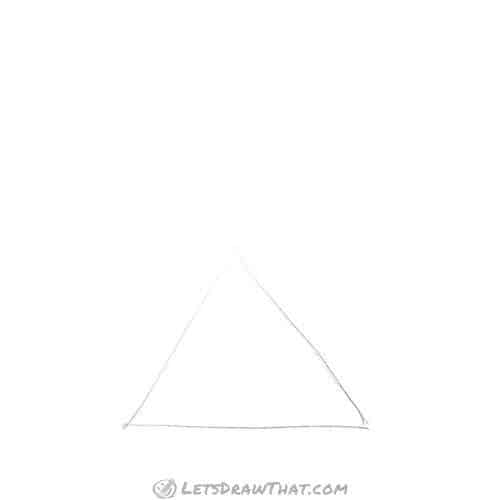So let’s draw the first triangle. As mentioned, all three sides are the same length. The internal angle of a equilateral triangle is 60 degrees. That makes our triangle a nice and symmetrical pyramid shape.

#### Step 2: Add two more upside down triangles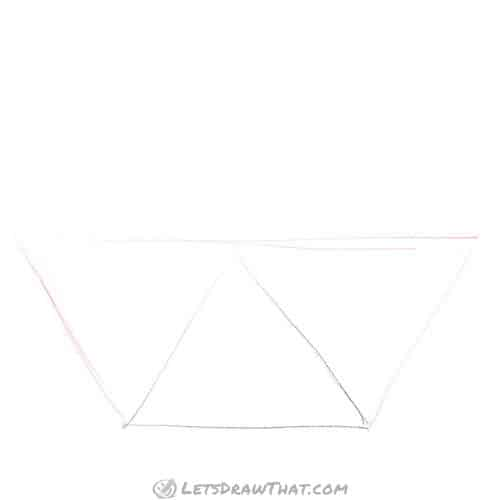Next we add two more triangles of the same size and shape . These will be drawn upside down, one on each side of our starting triangle.

Probably the easiest way is to draw a horizontal line through the top of the starting triangle. Then extend the sides, until they meet with the horizontal line.

Try to mirror the triangles as close as possible to the original – size and all angles.

#### Step 3: And two more triangles on top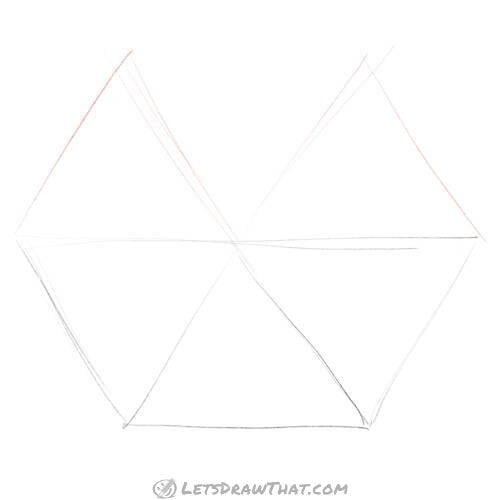Let’s add two more triangles – by now you are getting professional at drawing equilateral triangles.

These two stand back on the flat side, with their points up again, same as our first triangle.

The way to draw these is to extend the side lines of our first triangle past the centre of the hexagon. Then draw the outer sides. Again, try to mirror the bottom triangles as closely as possible.

As you can see, after I drew the outer sides, I had to adjust the inner ones a bit. Now the top points of my triangles line up nicely with the mirror triangles at the bottom half of the hexagon.

#### Step 4: Complete the hexagonWell, this is the easy bit. To close the last triangle, we just add a simple line at the top. That completes the hexagon drawing.

### How to draw a hexagon by hand: finished drawing

#### Completed hand-drawn hexagon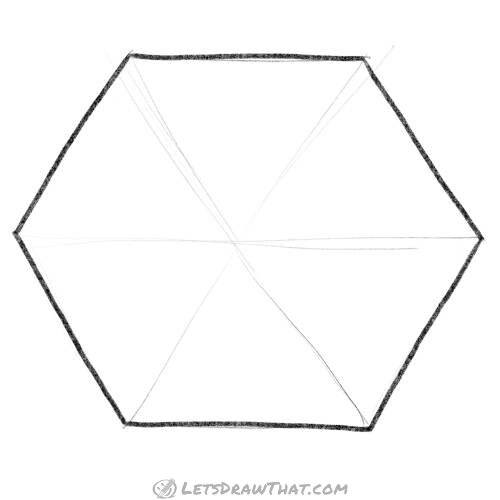Finally, let’s outline our hexagon. You can erase the triangle guidelines if you plan to use your hexagon in some further drawing.

As you can see, while the shape is not as perfect as the one constructed with the compass, we have still drawn a pretty regular hexagonal shape just by hand.

And if you feel like practising your hexagons on some real drawing, how about drawing a turtle? The entire turtle shell is made of hexagons!

Like this tutorial? Share it with others:
Here is another shape to construct next: How to draw a star: the easy and the fun way#### How to draw a star: the easy and the fun way

Learn how to draw a star two easy ways. Draw a nice symmetrical star using the letter "A" shape. And have fun drawing many wonky stars with just a single line.

## Love drawing? Never miss a tutorial!

Get notified when there is a new tutorial published on Let's Draw That! (2-4 times a month)
Tutorials by category:
Like this? Share the fun with others: